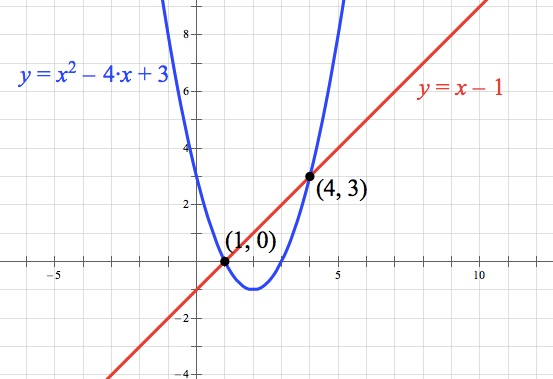PSAT #2, Section 4, #29

I’d get this one by substituting. Since both equations are given to you in y = notation, set them equal to each other.That tells you that x is either 1 or 4, so pick one and solve for what y must be in that case. Use the second equation because it’s simpler! For example, if x = 1, then the second equation tells you that y = 1 – 1 = 0. So in that case, the product of x and y is (1)(0) = 0.

The other possibility is that x = 4 and y = 3. In that case, the product of x and y would be (4)(3) = 12.

Note that although my first instinct here was to solve algebraically, this is also a good one to use your graphing calculator (if you have one). Just graph both equations, find either of the intersections, and multiply their coordinates to find the product of x and y.